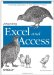# B.2. Loops

 < Day Day Up >

There are several types of loops that you can use in VBA. Here are three that I use most often.

#### B.2.1. For...Next Loop

The For...Next loop is used to go through a set of numbers and execute a block of code through each iteration. By default, VBA increments by 1 each time it comes to a Next statement. However, you can use Step to change the increment. The following example goes from 0 to 10 in increments of 2 and writes the value of the number to the Immediate Window:

` Dim x As Integer For x = 0 To 10 Step 2   Debug.Print x Next x `

#### B.2.2. For Each...Next Loop

This loop cycles through an array or collection. To use this loop, the variable that you use either has to be a type Variant, Object, or a specific type of object (such as Excel.Worksheet). Following is an example of cycling through each item in a collection using a Variant:

` Dim xColl As Collection Dim xItm As Variant   Set xColl = New Collection   xColl.Add 2 xColl.Add 10 xColl.Add 15   For Each xItm In xColl   Debug.Print xItm Next   Set xColl = Nothing `

There were several examples in the book of cycling through a collection of objects. Here is an example that you can use in Excel that puts the name of each worksheet and chart sheet in the active workbook into the Immediate Window.

` Dim xlWs As Excel.Worksheet Dim xlCs As Excel.Chart   For Each xlWs In ActiveWorkbook.Worksheets   Debug.Print xlWs.Name & " - Worksheet" Next xlWs For Each xlCs In ActiveWorkbook.Charts   Debug.Print xlCs.Name & " - Chart Sheet" Next xlCs   Set xlWs = Nothing Set xlCs = Nothing `

#### B.2.3. While Loop

The While loop continues to run a block of code until a condition is met. In the book, you saw this type of loop with a recordset where the code ran until it reached the end of the recordset. I find it interesting to learn more than one way to do something. Here is an example of using a While loop, which performs the same function as the previous For...Next loop above. Also, note that you end a While loop with Wend.

` Dim x As Integer x = 0 While x <= 10   Debug.Print x   x = x + 2 WendAddIns `

Loops are one of the most basic features of VBA, and they are critical to performing many automation tasks. There are many examples of loops in the VBA help that comes with Microsoft Office.

 < Day Day Up >Integrating Excel and Access
ISBN: 0596009739
EAN: 2147483647
Year: 2005
Pages: 132

Similar book on Amazon